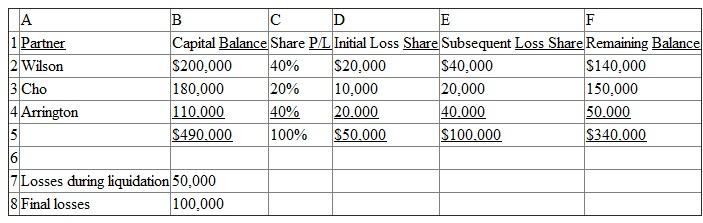# Quiz 15: Partnerships: Termination and Liquidation

There are a number of different ways that a spreadsheet could be created to solve this particular problem. Here is one possible approach: -Create Column Headings: In Cell A1, enter label text "Partner". In Cell B1, enter label text "Capital Balance". In Cell C1, enter label text "Share P/L". In Cell D1, enter label text "Initial Loss Share". In Cell E1, enter label text "Subsequent Loss Share". In Cell F1, enter label text "Remaining Balance". -Enter Account Information for each partner: In Cell A2, enter label text "Wilson." In Cell B2, enter Wilson's Capital Balance of \$200,000 and, in Cell C2, enter 40% as share of profit and loss. In Cell A3, enter label text "Cho." In Cell B3, enter Cho's Capital Balance of \$180,000 and, in Cell C3, enter 20% as share of profit and loss. In Cell A4, enter label text "Arrington." In Cell B4, enter Arrington's Capital Balance of \$110,000 and, in Cell C4, enter 40% as share of profit and loss. -Enter the amounts on which to base the calculations for each partner: In Cell A7, enter label text "Losses during liquidation" and, in Cell B7, enter the amount of \$50,000. In Cell A8, enter label text "Final Losses" and, in Cell B8, enter the amount of \$100,000. -Calculate Initial Loss Share: Multiply the "Losses during liquidation" amount by the percentage of "Share P/L" for each partner. To calculate the Initial Share Loss for Wilson, create the following formula in Cell D2: =+B7*C2. We need to also use this same general formula for both Cho and Arrington. However, if we drag the fill handle in Cell D2 into Cell D3 and D4, the reference to Cell B7 will automatically change to B8 and B9 respectively and the reference to Cell C2 will change to C3 and C4 respectively in order to adjust for the new cell position. The change to C3 and C4 is correct because those are the individual profit and loss percentages. No change, though, should be made to the reference to B7 because that is the overall loss in question. In order to "hold" the reference to Cell B7 when it is copied, we need to create what is known as an "ABSOLUTE" reference. Absolute references, which are cell references that always refer to cells in a specific location, can be created by placing a \$ symbol before the Column letter and/or the Row number. Thus, in Cell D2, change the formula to read =\$B\$7*C2, and then copy this formula to cells D3 and D4. The resulting formula in Cell D3 will be =\$B\$7*C3 and in Cell D4 it will be =\$B\$7*C4. The location of the reference to Cell B7 does not change due to the \$ symbol in front of the B and in front of the 7. -Calculate the Partners' Share of any Subsequent Losses: Repeat the same process as above, creating a formula in Cell E2 as follows: =+\$B\$8*C2 Copy this formula to Cells E3 and E4. -Calculate the Remaining Capital Balance: To calculate the Remaining Capital Balance, the beginning Capital Balance must be reduced by the Initial Loss Share and Subsequent Loss Share. In creating this last formula, it is important to note that the losses should be added together and then subtracted in total from the beginning capital balance. Therefore, enter the following function in Cell F2: =+B2-(D2+E2). The computation inside the parenthesis is performed first and then subtracted from the beginning capital balance (B2). Copy this formula to Cells F3 and F4 to complete the worksheet. Note that the use of the \$ is not used here because we do want B2, D2, and E2 to adjust to the new position when copied. Once this spreadsheet has been created, any of the variables may be changed and the results will adjust automatically. There are eight variables that can be changed: B2, B3, B4, B7, B8, C2, C3, and C4. C2, C3,and C4 must always add to 100%. Spreadsheet to Determine the Remaining Capital Balances for Wilson, Cho, and Arrington